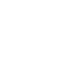Menu## What is the volumetric weight? And how do I calculate it?

The volumetric weight indicates the amount of cargo space a parcel takes up and is calculated for DPD Express parcels, which are transported by airfreight. The volumetric weight is only calculated if it exceeds the actual weight. You can calculate the volumetric weight conveniently in the DPD volumetric weight calculator. Or with the formula: Length (cm) x Width (cm) x Height (cm)/5,000 (cm³/kg).